JSL Syntax Reference > JSL Functions > Discrete Probability Functions
Publication date: 08/13/2020

# Discrete Probability Functions

Beta Binomial Distribution(k, p, n, delta)

Description

Returns the cumulative distribution function (cdf) of the beta binomial distribution. This is the probability that a beta binomially distributed random variable is less than or equal to k. The cdf is calculated as the summation of the beta binomial pmf for values of X from 0 to k.

Arguments

k

The count of interest. k must be an integer.

p

The probability of success for each trial, which must be between 0 and 1.

n

The number of trials, which must be greater than 1.

delta

The overdispersion parameter, which must be between Maximum[-p/(n-p-1), -(1-p)/(n-2+p)] and 1. When the overdispersion parameter is zero, the distribution reduces to Binomial(n, p).

Beta Binomial Probability(k, p, n, delta)

Description

Returns the probability mass function (pmf) of the beta binomial distribution. This is the probability that a beta binomially distributed random variable is equal to k. The pmf is parameterized as follows: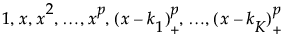Arguments

k

The count of interest. k must be an integer.

p

The probability of success for each trial, which must be between 0 and 1.

n

The number of trials, which must be greater than 1.

delta

The overdispersion parameter δ, which must be between Maximum[-p/(n-p-1), -(1-p)/(n-2+p)] and 1. When the overdispersion parameter is zero, the distribution reduces to Binomial(n, p).

Notes

The beta binomial distribution results from assuming that X|π follows a Binomial(n,π) distribution and π follows a Beta(p(1-δ)/δ,(1-p)(1-δ)/δ) distribution. It is useful when the data are a combination of several Binomial distributions that each have different probabilities of success. See Distributions in Basic Analysis.

Beta Binomial Quantile(p, n, delta, cumprob)

Description

Returns the smallest integer quantile for which the cumulative probability of the Beta Binomial(p, n, delta) distribution is larger than or equal to cumprob.

Arguments

p

The probability of success for each trial. p must be between 0 and 1.

n

The number of trials, which must be greater than 1.

delta

The overdispersion parameter δ, which must be between Maximum[-p/(n-p-1), -(1-p)/(n-2+p)] and 1. When the overdispersion parameter is zero, the distribution reduces to Binomial(n, p).

cumprob

The cumulative probability of the quantile desired. cumprob must be between 0 and 1.

Binomial Distribution(p, n, k)

Description

Returns the cumulative distribution function (cdf) of the binomial distribution. This is the probability that a binomially distributed random variable is less than or equal to k. The cdf is calculated as the summation of the binomial pmf for values of X from 0 to k.

Arguments

p

The probability of success for each trial. p must be between 0 and 1.

n

The number of trials.

k

The number of successes, which must be less than or equal to n.

Binomial Probability(p, n, k)

Description

Returns the probability mass function (pmf) of the binomial distribution. This is the probability that a binomially distributed variable is equal to k. The pmf is parameterized as follows: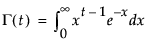Arguments

p

The probability of success for each trial. p must be between 0 and 1.

n

The number of trials.

k

The number of successes, which must be less than or equal to n.

Binomial Quantile(p, n, cumprob)

Description

Returns the smallest integer quantile for which the cumulative probability of the Binomial(p, n) distribution is larger than or equal to cumprob.

Arguments

p

The probability of success for each trial. p must be between 0 and 1.

n

The number of trials.

cumprob

The cumulative probability of the quantile desired. cumprob must be between 0 and 1.

Gamma Poisson Distribution(k, lambda, sigma)

Description

Returns the cumulative distribution function (cdf) of the gamma-Poisson distribution. This is the probability that a gamma-Poisson distributed random variable is less than or equal to k. The cdf is calculated as the summation of the gamma-Poisson pmf for values of X from 0 to k.

Arguments

k

The count of interest. k must be an integer.

lambda

The shape parameter λ, which much be greater than 0. This is the mean of the distribution.

sigma

The overdispersion parameter σ, which must be greater than or equal to 1. When the overdispersion parameter is 1, the distribution reduces to a Poisson(λ) distribution.

Gamma Poisson Probability(k, lambda, sigma)

Description

Returns the probability mass function (pmf) of the gamma-Poisson distribution. This is the probability that a gamma-Poisson distributed random variable is equal to k. The pmf is parameterized as follows: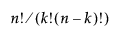where Γ(·) is the Gamma function.

Arguments

k

The count of interest. k must be an integer.

lambda

The shape parameter λ, which much be greater than 0. This is the mean of the distribution.

sigma

The overdispersion parameter σ, which must be greater than or equal to 1. When the overdispersion parameter is 1, the distribution reduces to a Poisson(λ) distribution.

Notes

The gamma Poisson distribution results from assuming that X|μ follows a Poisson(μ) distribution and μ follows a Gamma(λ/(σ-1),σ-1) distribution. It is useful when the data are a combination of several Poisson(μ) distributions that each have different values of μ. See Distributions in Basic Analysis.

Gamma Poisson Quantile(lambda, sigma, cumprob)

Description

Returns the smallest integer quantile for which the cumulative probability of the Gamma Poisson(lambda, sigma) distribution is larger than or equal to cumprob.

Arguments

lambda

The shape parameter λ, which much be greater than 0. This is the mean of the distribution.

sigma

The overdispersion parameter σ, which must be greater than or equal to 1. When the overdispersion parameter is 1, the distribution reduces to a Poisson(λ) distribution.

cumprob

The cumulative probability of the quantile desired. cumprob must be between 0 and 1.

Hypergeometric Distribution(N, K, n, x, <r>)

Description

Returns the cumulative distribution function (cdf) of the hypergeometric distribution. This is the probability that a hypergeometrically distributed random variable is less than or equal to x. The cdf is calculated as the summation of the hypergeometric pmf for values of X from 0 to x.

Arguments

N

The population size.

k

The number of items in the category of interest.

n

The sample size.

x

The count of interest, which must be less than or equal to n and k.

r

The optional odds ratio.

Hypergeometric Probability(N, k, n, x, <r>)

Description

Returns the probability mass function (pmf) of the hypergeometric distribution. This is the probability that a hypergeometrically distributed random variable is equal to x. The pmf is parameterized as follows: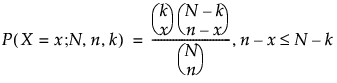Arguments

N

The population size.

k

The number of items in the category of interest.

n

The sample size.

x

The count of interest, which must be less than or equal to n and k.

r

The optional odds ratio.

Neg Binomial Distribution(p, n, k)

Description

Returns the cumulative distribution function (cdf) of the negative binomial distribution. This is the probability that a negative binomially distributed random variable is less than or equal to k. The cdf is calculated as the summation of the negative binomial pmf for values of X from 0 to k.

Arguments

p

The probability of success for each trial. p must be between 0 and 1.

n

The number of successes.

k

The number of failures before the nth success.

Neg Binomial Probability(p, n, k)

Description

Returns the probability mass function (pmf) of the negative binomial distribution. This is the probability that a negative binomially distributed random variable is equal to k. The pmf is parameterized as follows: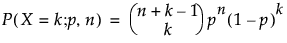Arguments

p

The probability of success for each trial. p must be between 0 and 1.

n

The number of successes.

k

The number of failures before the nth success.

Notes

The return value of the pmf is the probability of observing the nth success after k failures have occurred.

Poisson Distribution(lambda, k)

Description

Returns the cumulative distribution function (cdf) of the Poisson distribution. This is the probability that a Poisson distributed random variable with mean lambda is less than or equal to k. The cdf is calculated as the summation of the Poisson pmf for values of X from 0 to k.

Arguments

k

The number of events in a given time interval. k must be an integer.

lambda

The shape parameter λ, which much be greater than 0. This is the mean of the distribution.

Poisson Probability(lambda, k)

Description

Returns the probability mass function (pmf) of the Poisson distribution. This is the probability that a Poisson distributed random variable with mean lambda is equal to k. The pmf is parameterized as follows: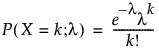Arguments

k

The number of events in a given time interval. k must be an integer.

lambda

The shape parameter λ, which must be greater than 0. This is the mean of the distribution.

Poisson Quantile(lambda, cumprob)

Description

Returns the smallest integer quantile for which the cumulative probability of the Poisson(lambda) distribution is larger than or equal to cumprob.

Arguments

lambda

The shape parameter λ, which must be greater than 0. This is the mean of the distribution.

cumprob

The cumulative probability of the quantile desired. cumprob must be between 0 and 1.